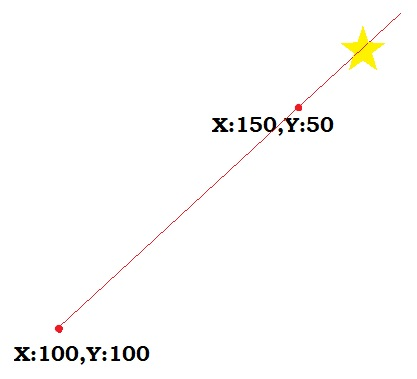# Move sprite from origin through a point

## Recommended Posts

Hello. I have searched for a way to move a sprite from an X,Y origin through and through another X,Y point but I can't seem to find a good example.

I have found examples that tween a sprite from one point to another, that move a sprite to the mouse pointer, and so on. But I cannot find the best way to start a sprite at X,Y and move it THROUGH a 2nd X,Y so that it keeps going past that point but stays at that angle.

Thank you!

EDIT:::

I have a feeling the solution is  velocityFromAngle or velocityFromRotation ... am I warm?

EDIT::::
Here's a pic describing what I am trying to accomplish##### Share on other sites

To find angle between points

``````dx = (endX - startX);
dy = (endY - startY);
tempAngle = Math.atan((dy)/(dx))*180/Math.PI;
movingStar.angle = tempAngle;``````

To move star towards its angle

``````movingStar.x += ((Math.cos((Math.PI*(movingStar.angle-180))/180) * movingStar.varSpeed));
movingStar.y += ((Math.sin((Math.PI*(movingStar.angle-180))/180) * movingStar.varSpeed));``````

##### Share on other sites

jjwallace is doing it the correct way.

Here's the lazy way: Phaser.Arcade.accelerateToXY(sprite, x, y, xSpeedMax, ySpeedMax).

( ;

##### Share on other sites

55 minutes ago, jjwallace said:

To find angle between points

```
```dx = (endX - startX);
dy = (endY - startY);
tempAngle = Math.atan((dy)/(dx))*180/Math.PI;
movingStar.angle = tempAngle;``````

To move star towards its angle

```
```movingStar.x += ((Math.cos((Math.PI*(movingStar.angle-180))/180) * movingStar.varSpeed));
movingStar.y += ((Math.sin((Math.PI*(movingStar.angle-180))/180) * movingStar.varSpeed));``````

Thank you very much!
when i use and console.log tempAngle I always get a result of ~40.   ???? I checked my start and end coordinates and they look just fine.

`dx = (endX - startX); dy = (endY - startY); tempAngle = Math.atan((dy)/(dx))*180/Math.PI;`I was using::: var angleDeg = Math.atan(endY - startY, endX - startX) * 180 / Math.PI;  but always resulted in ~ -90   ????

Aside from those issues, is it ok to change

`movingStar.x += ((Math.cos(...`TO

`movingStar.body.velocity.x = ((Math.cos(...`I dont have this looping to  update each position with a += so the velocity would get the object moving without the loop.

##### Share on other sites

56 minutes ago, drhayes said:

jjwallace is doing it the correct way.

Here's the lazy way: Phaser.Arcade.accelerateToXY(sprite, x, y, xSpeedMax, ySpeedMax).

( ;

I tried to multiquote these 2 but ...

Thank you! I tried using this but received an error:

Uncaught TypeError: Cannot read property 'accelerateToXY' of undefined

##### Share on other sites

Sorry about that, should've been more clear: "this.game.physics.arcade.accelerateToXY" is the call you'd use.

## Join the conversation

You can post now and register later. If you have an account, sign in now to post with your account.
Note: Your post will require moderator approval before it will be visible.×   Pasted as rich text.   Paste as plain text instead

Only 75 emoji are allowed.

×   Your previous content has been restored.   Clear editor

×   You cannot paste images directly. Upload or insert images from URL.